# NEET Physics Magnetism and Matter Questions Solved

A bar magnet of length l and magnetic dipole moment M is bent to form an arc which subtends an angle of $120°$ at centre. The new magnetic dipole moment will be

1.  $\frac{3\mathrm{M}}{2\mathrm{\pi }}$

2.  $\frac{3\sqrt{3}\mathrm{M}}{2\mathrm{\pi }}$

3.  $\frac{3\mathrm{M}}{\mathrm{\pi }}$

4.  $\frac{2\mathrm{M}}{\mathrm{\pi }}$

Complete Question Bank + Test Series
Complete Question Bank

Difficulty Level:

Complete Question Bank + Test Series
Complete Question Bank

Difficulty Level: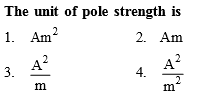Complete Question Bank + Test Series
Complete Question Bank

Difficulty Level: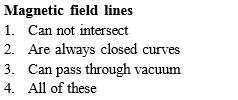Complete Question Bank + Test Series
Complete Question Bank

Difficulty Level: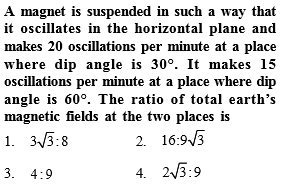Complete Question Bank + Test Series
Complete Question Bank

Difficulty Level:

1.

2.

3.

4.  ${\mathrm{tan}}^{-1}\left(\frac{2}{\sqrt{3}}\right)$

Complete Question Bank + Test Series
Complete Question Bank

Difficulty Level: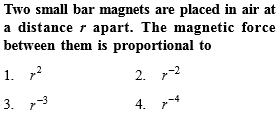Complete Question Bank + Test Series
Complete Question Bank

Difficulty Level: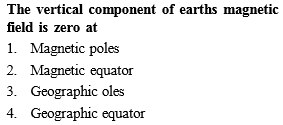Complete Question Bank + Test Series
Complete Question Bank

Difficulty Level: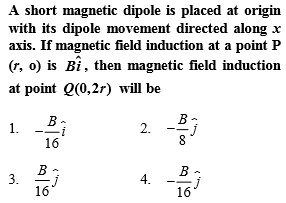Complete Question Bank + Test Series
Complete Question Bank

Difficulty Level:

The magnetic field at a point x on the axis of a small bar magnet is equal to the field at a point y on the equator of the same magnet. The ratio of the distances of x and y from the centre of the magnet is
(a) ${2}^{-3}$

(b) ${2}^{-1}{3}}$

(c) ${2}^{3}$

(d) ${2}^{1}{3}}$